JEE## Current Electricity Important Concepts for JEE

The chapter Current Electricity is a continuation of the concepts related to the electric current covered in the previous classes and consists of s

### Important Topics of Current Electricity

• Electric current density and drift velocity

• Ohm’s law and temperature depend on resistivity.

• Grouping of Resistors

• Colour code of carbon resistor

• Grouping of cells

• Kirchhoff’s Law

• Wheatstone bridge

• Metre bridge

• Potentiometer

### Solved Examples

1. The internal resistance of a cell of emf 2V is 0.1 Ω. It is connected to a resistance of 3.9 Ω. Find the voltage across the cell.

Sol: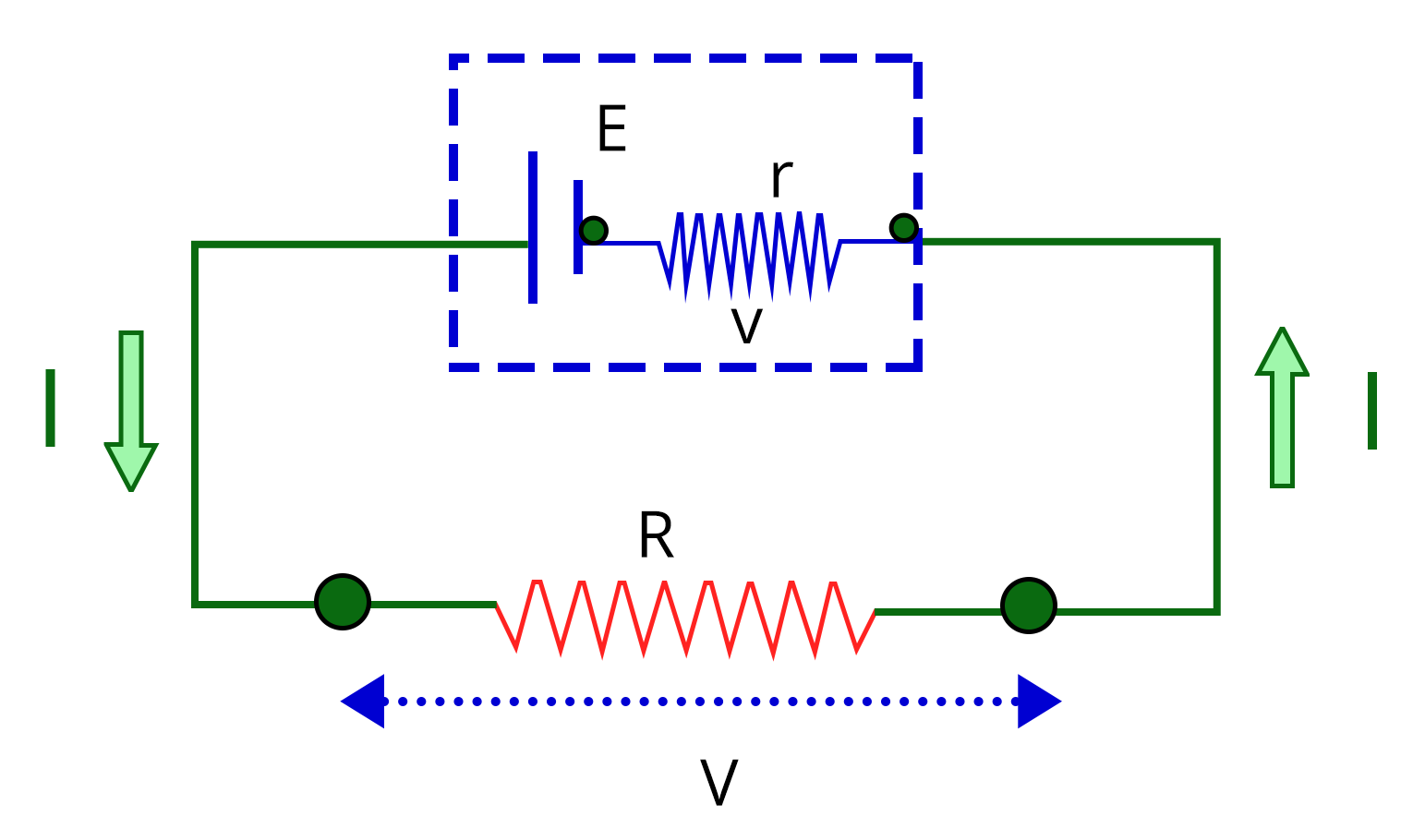Given:

Emf of the cell, E = 2V

Internal resistance of the cell, r = 0.1 Ω

Resistance connected to the cell, R = 3.9 Ω

Let V be the terminal voltage across the cell.

We know that the voltage drop across the resistor,

$V=IR$....(1)

Applying Kirchhoff's voltage law,

$E-V-Ir=0$

$E-IR-Ir=0$

$2V-3.9I-0.1I=0$

$I=\dfrac{2}{4}=0.5~A$

Substitute the value of current I in equation (1) to obtain the voltage across the cell V.

$V=0.5\times 3.9=1.95~V$

Therefore, the voltage across the cell is 1.95 V

Key point: Since resistor R and the cell are in parallel connection, the voltage across them are equal.

2. The resistance of a wire is R ohm. If it is melted and stretched to n times its original length, its new resistance will be_________.

Sol:

Given:

The resistance of the wire is R.

Let the length and area of the cross-section of wire be l and A, respectively and the resistivity of the wire be 𝜌.

If the length of the wire is stretched to n times, then the area of the cross-section of the wire is reduced by n times.

Let the length and area of the cross-section of the stretched wire be l’ and A’, respectively.

The resistance of the stretched wire is given by

$R'=\rho\dfrac{l'}{A'}$

$R'=\rho\dfrac{(nl)}{\left(\dfrac{A}{n}\right)}$

$R'=\rho\dfrac{n^2l}{A}$

$R'=n^2\times\left(\rho\dfrac{l}{A}\right)$

$R'=n^2R$

Therefore, the resistance of the stretched wire is n2 times the initial resistance.

Key point: When a wire is stretched, its length increases and the area of cross-section decreases while keeping the volume constant.

### Previous Years’ Questions from JEE Paper

1. Five equal resistances are connected in a network as shown in the figure. The net resistance between points A and B is______. (Feb -2021)

a. 3R/2

b. R/2

c. R

d. 2R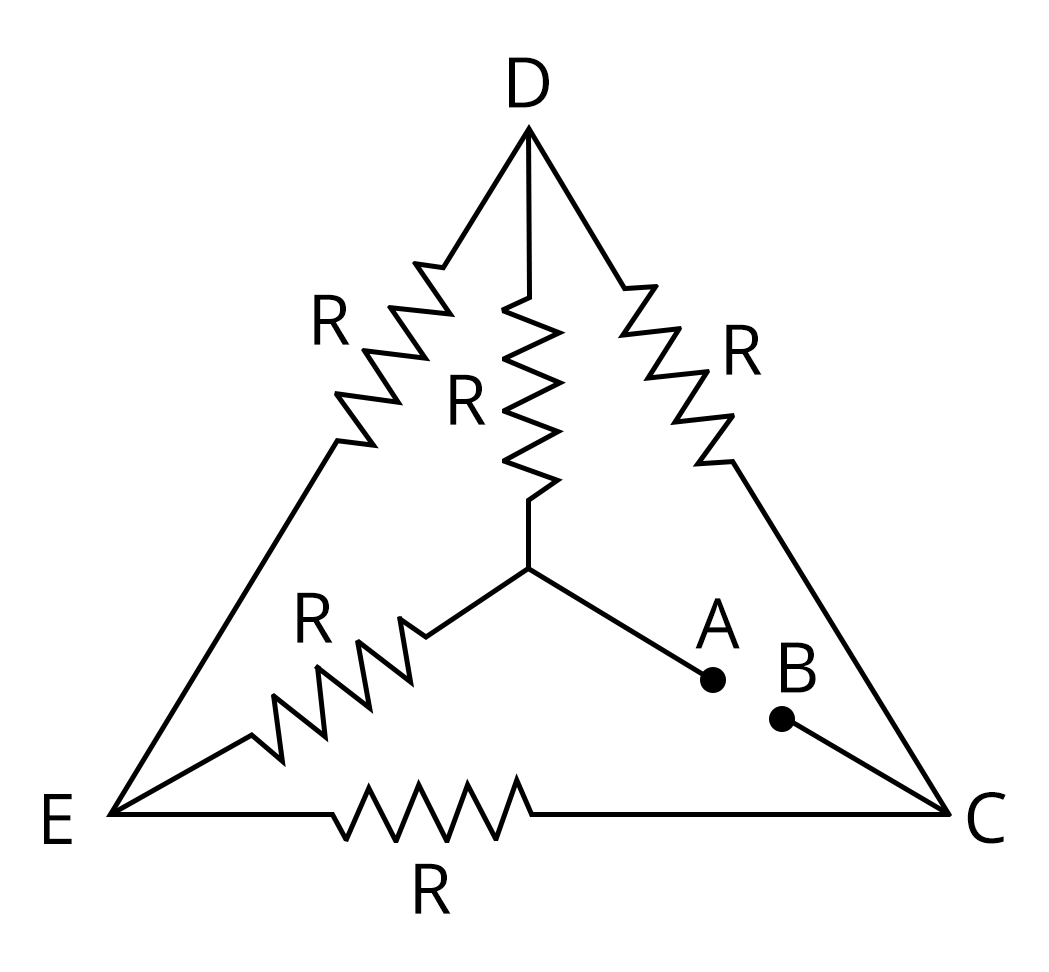Sol:

If you observe the diagram closely, you can see that the above circuit diagram is a balanced Wheatstone bridge since all the resistances are the same. Therefore, it satisfies the condition

$\dfrac{R_2}{R_{1}}=\dfrac{R_4}{R_3}$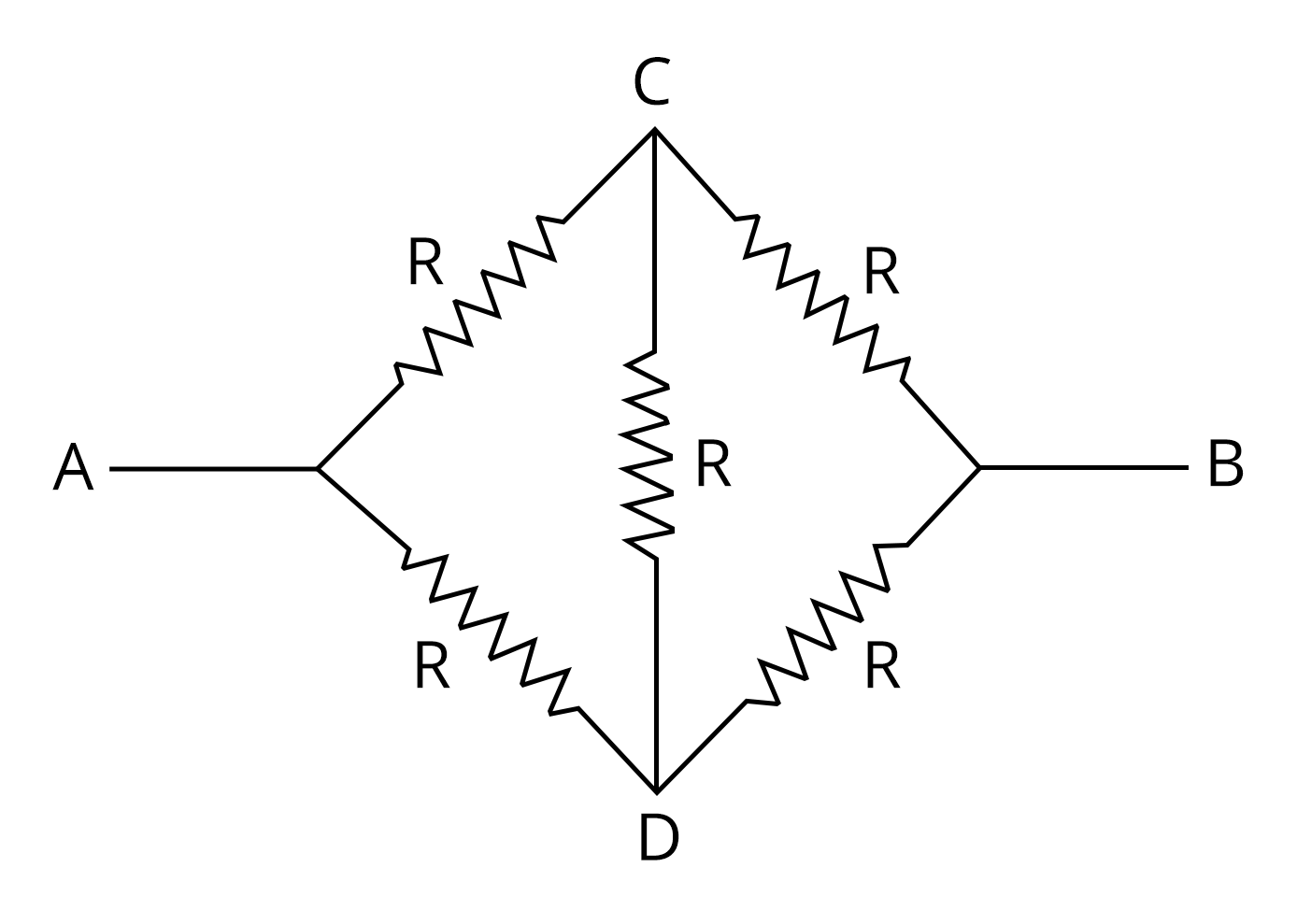Since it is balanced, the current does not pass through the resistor in the bridge and can be removed while calculating the effective resistance.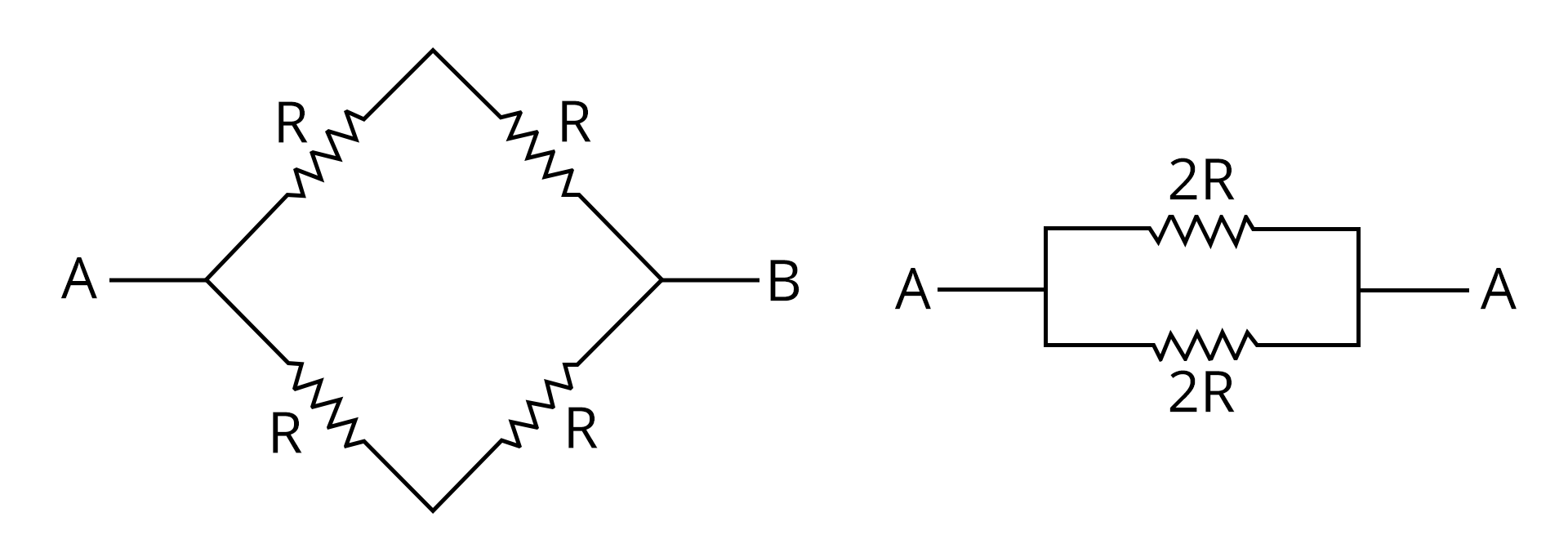The two 2R resistors are in parallel, so the equivalent resistance between A and B is

$R_{eq}=\dfrac{2R\times 2R}{2R+2R}$

$R_{eq}=\dfrac{4R^2}{4R}$

$R_{eq}=R$

Therefore, the equivalent resistance between A and B is R and the correct answer is option C.

Key point to remember: When a difficult circuit diagram having resistors are given, always look for the possibility of a balanced Wheatstone bridge.

2. The length of a potentiometer wire is 1200 𝑐𝑚 and it carries a current of 60 𝑚𝐴. For a cell of emf 5 𝑉 and internal resistance of 20Ω, the null point on it is found to be at 1000 𝑐𝑚. The resistance of the whole wire is_____. (JEE 2020)

a. 80Ω

b. 100Ω

c. 120Ω

d. 60Ω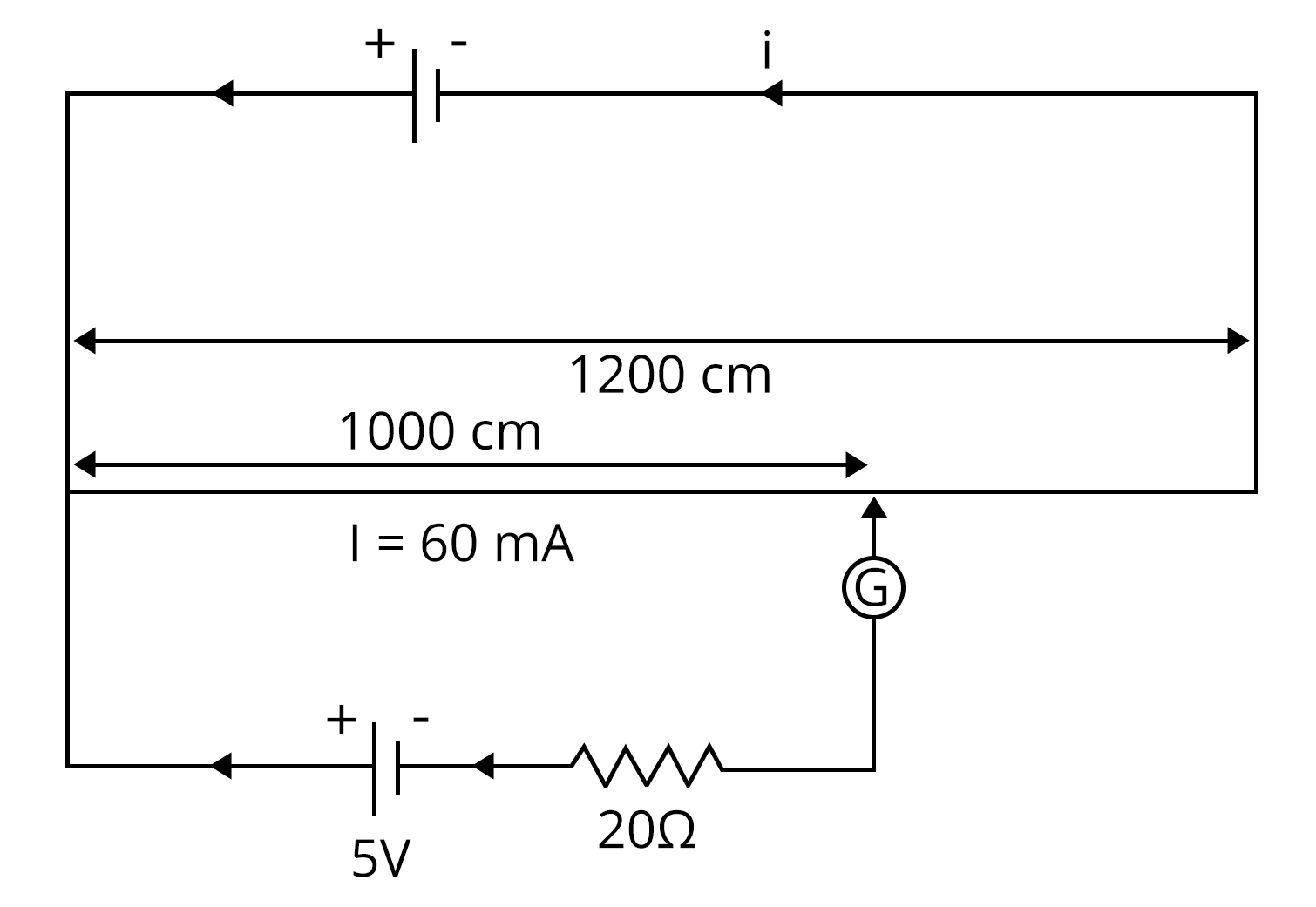Sol:

Given:

The null point of the potentiometer = 1000 cm

The current through the potentiometer wire, I = 60 mA

Emf of the cell in the secondary circuit, E = 5 V

At the null point, no current passes through the galvanometer and hence no current is drawn from the cell. Therefore, the emf of the cell is equal to the potential difference across the section of the wire having a length of 1000 cm.

So, the resistance of the wire of 1000 cm (R1) can be calculated.

$R_1=\dfrac{E}{I}$

$R_1=\dfrac{5~V}{60~\text{mA}}$

$R_1=83.33~ \Omega$

Let the resistance of the wire per unit length be r.

$r=\dfrac{83.33~ \Omega}{1000~\text{cm} }$

$r=83.33\times 10^{-3}~ \Omega/cm$

Now, the resistance of the 1200 cm potentiometer wire (R) is obtained by

$R=83.33\times 10^{-3}~ \Omega/cm~\times 1200~\text{cm}$

$R=100~\Omega$

Therefore, the correct answer is option B.

Key point to remember: At the balancing point, no current is drawn from the cell and hence the terminal voltage and emf are equal.

### Practice Questions

1. A uniform wire of resistance 9 Ω is cut into three equal parts. They are connected in the form of an equilateral  triangle ABC. A cell of emf 2V and negligible internal  resistance is connected across B and C. Potential difference across AB is____. (Ans: 1 V)

2. A filament bulb (500 W, 100V) is to be used in a 230 V main supply. When a resistance R is connected in series, it works perfectly and consumes 500 W. The value of R is_______. (Ans: 26 Ω)

### Conclusion

In this article, we have provided important information regarding the chapter on Current Electricity, such as important concepts, types of current electricity formulas, circuit problems, etc. More focus has to be given to circuit problems and measuring instruments like metre bridge and potentiometer to score high marks for JEE.

See MoreBook your Free Demo session
Get a flavour of LIVE classes here at VedantuVedantu Improvement Promise
We promise improvement in marks or get your fees back. T&C Apply*All
See All## Toppers## Master TeachersFrom IITs & other top-tier colleges with 5+ years of experienceYou can count on our specially-trained teachers to bring out the best in every student.They have taught over 4.5 crore hours to 10 lakh students in 1000+ cities in 57 countries11+ years exp

### Shreyas

Physics master teacher

#### NIT Nagpur4+ years exp

### Nidhi Sharma

Chemistry master teacher

#### Jamia Hamdard2+ years exp

### Luv Mehan

Chemistry Master Teacher

#### IISc BangaloreFAQ

1. What is the weightage of the current electricity in JEE?

Around 2-3 questions are asked in JEE from various parts of the chapter. Most of the questions are based on electric current example problems and questions can also be asked by linking them to concepts from other chapters.

2. What section has to be given more importance in the chapter on current electricity?

More focus must be laid on solving electric current example problems and getting the answer without committing any mistakes. Therefore, students should be able to solve any problems related to this chapter by practising more and more problems.

3. How to score good marks in JEE from the chapter on Current Electricity?

First of all, learn and understand the concepts and current electricity formulae in each chapter in depth. This should be followed by solving all the problems given in NCERT textbooks. Then go through the question papers of the last 20 years. Try to solve them and observe the pattern to score good marks in JEE.## Notice board

News
BlogTrending pages